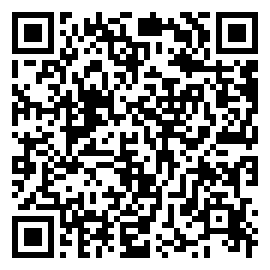Jimmy Zhang Apr 08, 2017
•$e^x$ 在导数题中的出镜率不可谓不低，而其最显著的特点便是：2010年全国大纲卷理科数学的压轴题是一道难题，并且此题在高考中坑完了一批无辜的学生还变着花样在模拟题中坑更多的学生，乃至于在不久前我甚至做到一道与该题几乎没有区别的2016武汉模拟题。在各大资料都倾向于使用令高中生头疼的放缩法亦或是普通高中生听都没听说过的洛必达法则（后文中也会介绍）解答时，我们来看看它与上述技巧能碰撞出怎样的火花：

1. (2010.全国大纲卷理) 设函数 $f(x) = 1 - e^{-x}$
(1) 证明：当 $x > -1$ 时，$f(x) \ge \frac{x}{x+1}$
(2) 设当 $x \ge 0$ 时，$f(x) \le \frac{x}{ax + 1}$，求 $a$ 的取值范围。

： (2)

\begin{aligned}
& f(x) \le \frac{x}{ax + 1} \
& \Leftrightarrow 1 - e^{-x} \le \frac{x}{ax + 1} \
& \Leftrightarrow 1 - \frac{x}{ax + 1} \le e^{-x} \
& \Leftrightarrow e^x(1 - \frac{x}{ax + 1}) \le 1
\end{aligned}

$F(x) = e^x(1 - \frac{x}{ax + 1}) - 1$

\begin{aligned}
\therefore F’(x) & = e^x[1 - \frac{x}{ax + 1} - \frac{(ax - 1) - ax}{(ax + 1)^2}] \
& = \frac{e^x}{(ax + 1)2}[(a2 - a)x^2 + (2a - 1)x] \
& = \frac{xe^x}{(ax + 1)2}[(a2 - a)x + (2a - 1)]
\end{aligned}

I.$a < 0$
$\therefore$$x \in (-\frac{1}{a},+\infty)$时：

$\frac{x}{ax + 1} < 0$

$\because$$x \in [0,+\infty)$时：

$f(x) = 1 - e^{-x} \ge 0$

$\therefore$$x \in (-\frac{1}{a},+\infty)$ 时：

$f(x) > \frac{x}{ax + 1}$

II.$a \in [0,\frac{1}{2}]$，有：

$\begin{cases}$
a^2 - a \le 0 \
2a - 1 \le 0
\end{cases}

$(a^2 - a)x + (2a - 1) \le 0$

$\therefore F’(x) \le 0$

$\therefore F(x)$$[0,+\infty)$ 单调递减 ，故：

$\therefore F(x) \le F(0) = 0$

III.$a \in (\frac{1}{2}, 1)$，有：

$\begin{cases}$
a^2 - a \le 0 \
2a - 1 > 0
\end{cases}

$f’(x) = 0$，有：

$x = \frac{1 - 2a}{a^2 - a} > 0$

$\therefore F(x)$$[0, \frac{1 - 2a}{a^2 - a}]$上单调递增，故：

$F(\frac{1 - 2a}{a^2 - a}) > F(0) = 0$

IV.$a \ge 1$

$\begin{cases}$
a^2 - a \ge 0 \
2a - 1 \ge 0
\end{cases}

$(a^2 - a)x + (2a - 1) > 0$

$\therefore F’(x) > 0$

$\therefore F(x)$$[0,+\infty)$ 单调递增，故：

$F(x) \ge F(0) = 0$

2011年全国大纲卷理科数学的压轴题也是一道有名的难题，让我们来看看优化函数又能起到怎样的效果。

2. (2011.全国大纲卷理) 已知函数$f(x) = \frac{alnx}{x+1} + \frac{b}{x}$，曲线 $y = f(x)$ 在点$(1, f(1))$ 处的切线方程为 $x + 2y - 3 = 0$
(1) 求 $a$, $b$ 的值；
(2) 如果当 $x > 0$，且 $x \neq 0$时，$f(x) > \frac{lnx}{x - 1} + \frac{k}{x}$，求 $k$ 的取值范围。

: (2)

$\frac{lnx}{x + 1} + \frac{1}{x} > \frac{lnx}{x - 1} + \frac{k}{x}$

$\Leftrightarrow \begin{cases}$
(k - 1)(x - \frac{1}{x}) + 2lnx < 0 & (x > 1) \
(k - 1)(x - \frac{1}{x}) + 2lnx > 0 & (0 < x < 1)
\end{cases}

$g(x) = (k - 1)(x - \frac{1}{x}) + 2lnx$

$\therefore 0 < x < 1$时，$g(x) > 0$$x > 1$时，$g(x) < 0$

$g’(x) = \frac{(k - 1)x^2 + 2x + k - 1}{x^2}$

$h(x) = (k - 1)x^2 + 2x + k - 1$

I.$k \le 0$
$h(x)$

$\Delta = 4 - 4(k - 1)^2 \le 0$

$h(x) \le 0$

$\therefore g’(x) \le 0$

$\therefore g(x)$$(0,1)$$(1,+\infty)$ 单调递减
$\therefore$$(0,1)$ 上，$g(x) > g(1) = 0$ 恒成立；在 $(1,+\infty)$ 上，$g(x) < g(1) = 0$ 恒成立
$\therefore$ 满足要求

II.$0 < k < 1$
$h(x)$

$\Delta = 4 - 4(k - 1)^2 > 0$

$h(x)$ 两零点分别为 $x_1$$x_2$，且 $x_2 > x_1$

$x_1 + x_2 = -\frac{2}{k - 1} > 2$

$\therefore x_2 > 1$

$\therefore$ 由二次函数性质，当 $x \in (1, x_2)$ 时有：

$g(x) > 0$

$\therefore$ 不符要求，舍去。

III.$k \ge 1$
$\therefore k - 1 \ge 0$

$\because$$(1,+\infty)$ 区间内 $x$ 单调递增，$\frac{1}{x}$ 单调递减
$\therefore x - \frac{1}{x}$ 在该区间内单调递增

$\therefore x - \frac{1}{x} > 1 - \frac{1}{1} = 0$

$\therefore$$(1,+\infty)$ 区间内：

$g(x) = (k - 1)(x - \frac{1}{x}) + 2lnx > 0$

$\therefore$ 不符要求，舍去.

$\therefore$ 综上，$k \in (-\infty,0]$

3. (2016.武汉模拟) 已知函数 $f(x) = e^x - ax^2$，曲线 $y = f(x)$$x = 1$ 处的切线方程为 $y = bx + 1$
(1) 求 $a$, $b$ 的值；
(2) 求函数 $f(x)$$[0,1]$ 上的最大值；
(3) 证明：当 $x > 0$ 时，$e^x + (1 - e)x - xlnx -1 \ge 0$.

(1) $a = 1$; $b = e - 2$
(2) 最大值即 $f(1) = e - 1$

$F(x) = \frac{e^x}{x} + (1 - e) - lnx - \frac{1}{x}$

\begin{aligned}
\therefore F’(x) & = \frac{xe^x - (e^x - 1)}{x^2} - \frac{1}{x} \
& = \frac{(x - 1)e^x - (x - 1)}{x^2} \
& = \frac{(x - 1)(e^x - 1)}{x^2}
\end{aligned}

$F’(x) = 0$，得 $x = 1$
$x \in (0,1)$ 时，$F’(x) < 0$$F(x)$单调递减；
$x \in (1,+\infty)$ 时，$F’(x) > 0$$F(x)$ 单调递增；
$\therefore F(1)$$F(x)$ 的极小值点

$\therefore F(x) \ge F(1) = 0$

$\therefore xF(x) \ge 0$

$\therefore$ 得证。

• 对于含 $e^x$ 的函数，我们应考虑将函数化为 $e^xg(x) + b$$e^{-x}g(x) + b$ 的形式。
• 对于含 $h(x)lng(x)$ 的函数，我们应考虑把函数化为 $k(x) + lng(x)$ 的形式。
• 对于两者都有的函数，一般不这样考虑，但在简单的情况下顾全一者即可求出。

• 张杨文主编.高考数学你真的掌握了吗 函数 [M].北京：清华大学出版社, 2014 (2016.5 重印)

This blog is under a CC BY-NC-SA 4.0 Unported License.